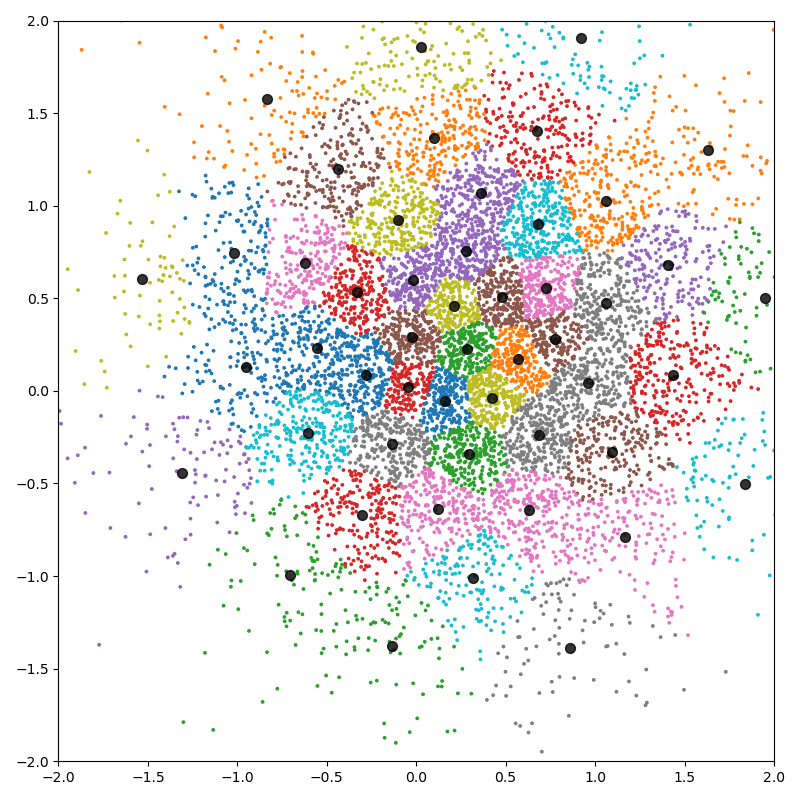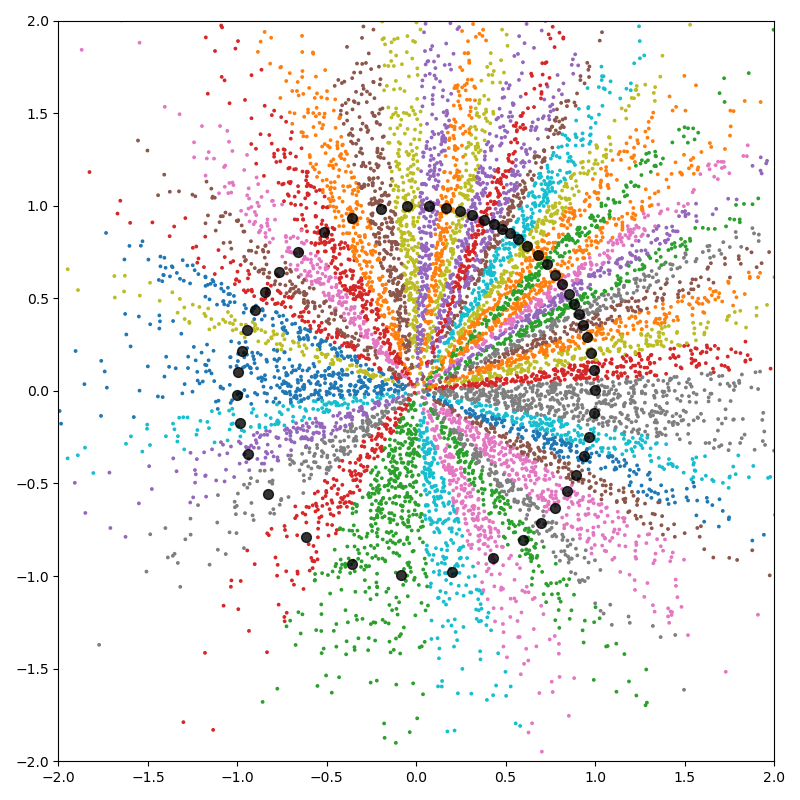# K-means clustering - PyTorch API

The `pykeops.torch.LazyTensor.argmin()` reduction supported by KeOps `pykeops.torch.LazyTensor` allows us to perform bruteforce nearest neighbor search with four lines of code. It can thus be used to implement a large-scale K-means clustering, without memory overflows.

Note

For large and high dimensional datasets, this script outperforms its NumPy counterpart as it avoids transfers between CPU (host) and GPU (device) memories.

## Setup

Standard imports:

```import time
import torch
from matplotlib import pyplot as plt
from pykeops.torch import LazyTensor

use_cuda = torch.cuda.is_available()
dtype = torch.float32 if use_cuda else torch.float64
device_id = "cuda:0" if use_cuda else "cpu"
```

Simple implementation of the K-means algorithm:

```def KMeans(x, K=10, Niter=10, verbose=True):
"""Implements Lloyd's algorithm for the Euclidean metric."""

start = time.time()
N, D = x.shape  # Number of samples, dimension of the ambient space

c = x[:K, :].clone()  # Simplistic initialization for the centroids

x_i = LazyTensor(x.view(N, 1, D))  # (N, 1, D) samples
c_j = LazyTensor(c.view(1, K, D))  # (1, K, D) centroids

# K-means loop:
# - x  is the (N, D) point cloud,
# - cl is the (N,) vector of class labels
# - c  is the (K, D) cloud of cluster centroids
for i in range(Niter):
# E step: assign points to the closest cluster -------------------------
D_ij = ((x_i - c_j) ** 2).sum(-1)  # (N, K) symbolic squared distances
cl = D_ij.argmin(dim=1).long().view(-1)  # Points -> Nearest cluster

# M step: update the centroids to the normalized cluster average: ------
# Compute the sum of points per cluster:
c.zero_()

# Divide by the number of points per cluster:
Ncl = torch.bincount(cl, minlength=K).type_as(c).view(K, 1)
c /= Ncl  # in-place division to compute the average

if verbose:  # Fancy display -----------------------------------------------
if use_cuda:
torch.cuda.synchronize()
end = time.time()
print(
f"K-means for the Euclidean metric with {N:,} points in dimension {D:,}, K = {K:,}:"
)
print(
"Timing for {} iterations: {:.5f}s = {} x {:.5f}s\n".format(
Niter, end - start, Niter, (end - start) / Niter
)
)

return cl, c
```

## K-means in 2D

First experiment with N=10,000 points in dimension D=2, with K=50 classes:

```N, D, K = 10000, 2, 50
```

Define our dataset:

```x = 0.7 * torch.randn(N, D, dtype=dtype, device=device_id) + 0.3
```

Perform the computation:

```cl, c = KMeans(x, K)
```
```K-means for the Euclidean metric with 10,000 points in dimension 2, K = 50:
Timing for 10 iterations: 0.01269s = 10 x 0.00127s
```

Fancy display:

```plt.figure(figsize=(8, 8))
plt.scatter(x[:, 0].cpu(), x[:, 1].cpu(), c=cl.cpu(), s=30000 / len(x), cmap="tab10")
plt.scatter(c[:, 0].cpu(), c[:, 1].cpu(), c="black", s=50, alpha=0.8)
plt.axis([-2, 2, -2, 2])
plt.tight_layout()
plt.show()
```KeOps is a versatile library: we can add support for the cosine similarity with a few lines of code.

```def KMeans_cosine(x, K=10, Niter=10, verbose=True):
"""Implements Lloyd's algorithm for the Cosine similarity metric."""

start = time.time()
N, D = x.shape  # Number of samples, dimension of the ambient space

c = x[:K, :].clone()  # Simplistic initialization for the centroids
# Normalize the centroids for the cosine similarity:
c = torch.nn.functional.normalize(c, dim=1, p=2)

x_i = LazyTensor(x.view(N, 1, D))  # (N, 1, D) samples
c_j = LazyTensor(c.view(1, K, D))  # (1, K, D) centroids

# K-means loop:
# - x  is the (N, D) point cloud,
# - cl is the (N,) vector of class labels
# - c  is the (K, D) cloud of cluster centroids
for i in range(Niter):
# E step: assign points to the closest cluster -------------------------
S_ij = x_i | c_j  # (N, K) symbolic Gram matrix of dot products
cl = S_ij.argmax(dim=1).long().view(-1)  # Points -> Nearest cluster

# M step: update the centroids to the normalized cluster average: ------
# Compute the sum of points per cluster:
c.zero_()

# Normalize the centroids, in place:
c[:] = torch.nn.functional.normalize(c, dim=1, p=2)

if verbose:  # Fancy display -----------------------------------------------
if use_cuda:
torch.cuda.synchronize()
end = time.time()
print(
f"K-means for the cosine similarity with {N:,} points in dimension {D:,}, K = {K:,}:"
)
print(
"Timing for {} iterations: {:.5f}s = {} x {:.5f}s\n".format(
Niter, end - start, Niter, (end - start) / Niter
)
)

return cl, c
```

Timings are similar to the Euclidean case:

```cl, c = KMeans_cosine(x, K)
```
```K-means for the cosine similarity with 10,000 points in dimension 2, K = 50:
Timing for 10 iterations: 0.00492s = 10 x 0.00049s
```

Clusters behave as slices around the origin:

```plt.figure(figsize=(8, 8))
plt.scatter(x[:, 0].cpu(), x[:, 1].cpu(), c=cl.cpu(), s=30000 / len(x), cmap="tab10")
plt.scatter(c[:, 0].cpu(), c[:, 1].cpu(), c="black", s=50, alpha=0.8)
plt.axis([-2, 2, -2, 2])
plt.tight_layout()
plt.show()
```## K-means in dimension 100

Second experiment with N=1,000,000 points in dimension D=100, with K=1,000 classes:

```if use_cuda:
N, D, K = 1000000, 100, 1000
x = torch.randn(N, D, dtype=dtype, device=device_id)
cl, c = KMeans(x, K)
cl, c = KMeans_cosine(x, K)
```
```K-means for the Euclidean metric with 1,000,000 points in dimension 100, K = 1,000:
Timing for 10 iterations: 0.56774s = 10 x 0.05677s

K-means for the cosine similarity with 1,000,000 points in dimension 100, K = 1,000:
Timing for 10 iterations: 0.30200s = 10 x 0.03020s
```

Total running time of the script: ( 0 minutes 1.504 seconds)

Gallery generated by Sphinx-Gallery